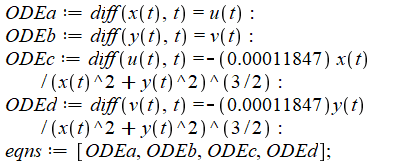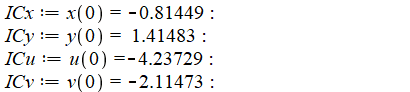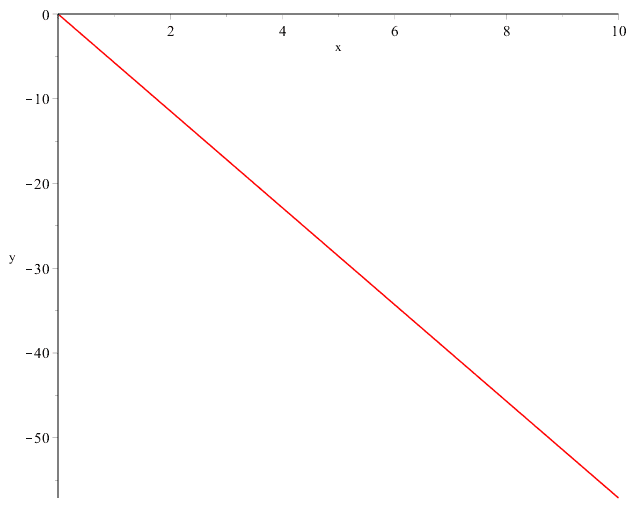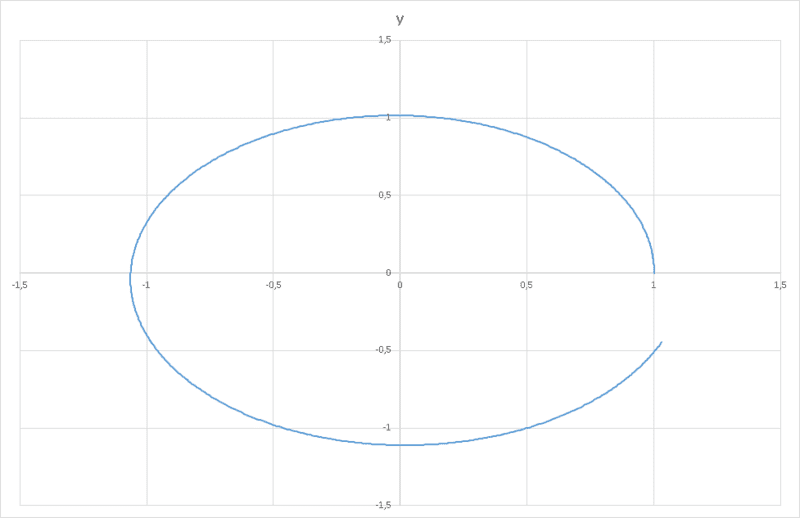# ODE System solution Maple

• Maple
• incredibe1999

#### incredibe1999

Hey there! I was trying to plot a ODE solution, but am not getting what I should be. It is actually plotting the orbit of Earth with Sun at the origin.
My equationsThe (-0.00011847) is GM.
The Initial Conditions:The plot I get:Should not I be getting a elliptic/circular plot as the orbital positions of the Earth? Also, here the period is t=1. The position is supposed to be same at t=0 and t=1. Help, please!

#### Attachments

What is the code you used to generate this plot?

What is the code you used to generate this plot?
I used the ODE Analyzer.

Well, that is not very helpful for us in finding out what goes wrong.

If I use your picture (please post something one can copy as text next time -- it avoids typos) but take different values, even good old Euler in Excel gets me a plot I expect:values:
Code:
dt 0,01
x_0 1
y_0 0
vx_0 0
vy_0 1
GM 1
so the expressions look OK (provided I reproduced them correctly)

Now: what are the units for your values (and for time) ?